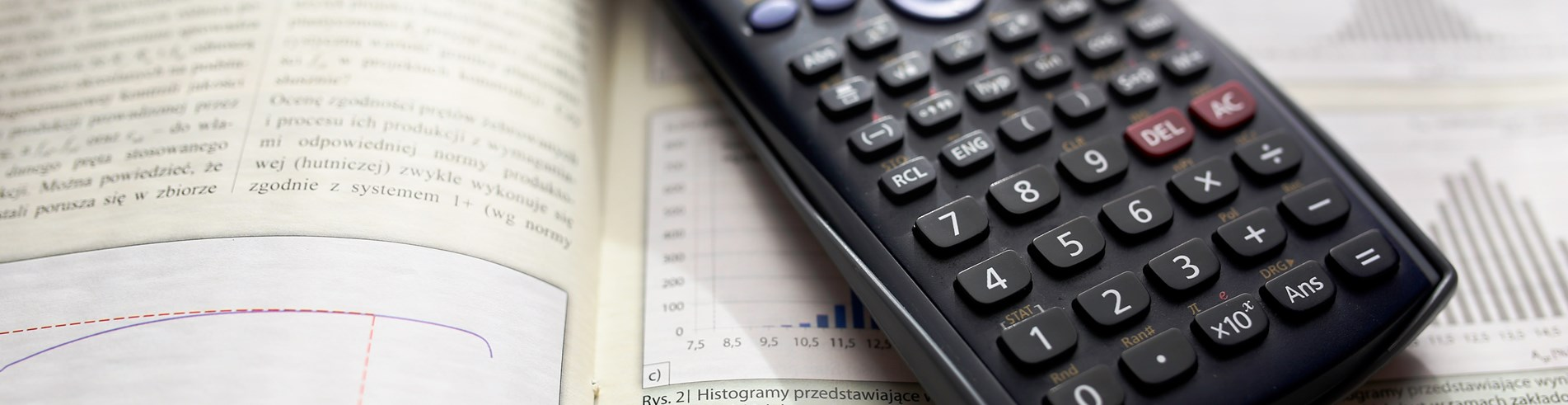Close# Further maths - A level

#### What will I learn?

You will study two further maths modules, FP1 and FP2, as well as four further applied modules, the choice of which depends on what you have already selected at A level. The further maths modules content includes:

• Complex numbers and coordinate systems
• Series, include finite, Maclaurin and Taylor and Matrix Algebra
• Numerical solutions of equations and proof by mathematical induction

The applied content depends on which combination of modules you choose from: Statistics and mechanics or decision maths. You will be expected to:

• use mathematical skills and knowledge to solve problems
• understand and demonstrate what is meant by proof in maths
• solve real life problems using mathematical logic and argument

#### What skills do I need?

Maths is rather different from many other subjects. An essential part is the challenge of analysing and solving a problem and the satisfaction and confidence gained from achieving a ‘correct’ answer. For students wishing to follow this course, a grade A (or higher) in Higher GCSE Maths is required.

#### How is the course graded?

You will take an extra six modules, each module is tested by a written examination. All units are equally weighted.

#### What is next for me after this course?

Like A level maths, the further maths course is a sought-after qualification for entry to a wide variety of full-time courses in higher education. Higher education courses or careers that require A level further maths are similar to that mentioned on the A level Maths option details.